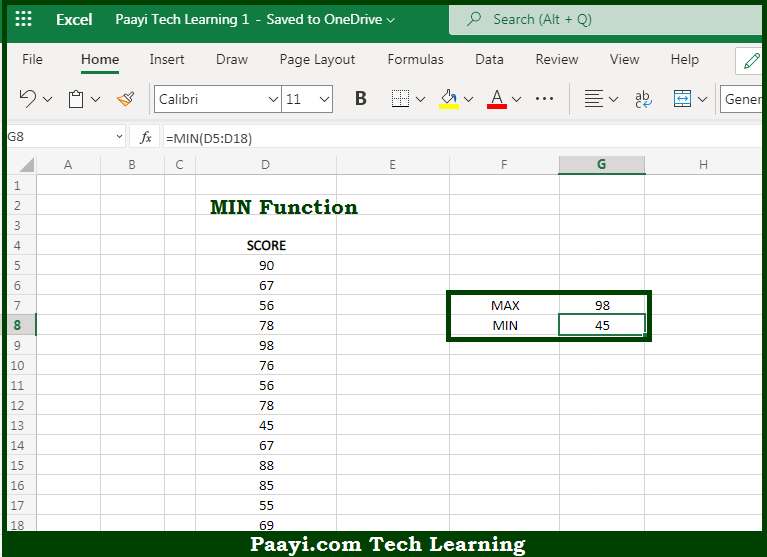# Learn How to Use Microsoft Excel MIN Function

Written by | 0 Comments | 523 Views

In this article, you will learn how to use the Microsoft Excel MIN function and its prime function in Microsoft Excel. You will also get to know the Microsoft Excel MIN function return value and syntax with the help of some examples.

Microsoft Excel MIN Function

The main purpose of the Microsoft Excel MIN function is to get the smallest value. That implies, with the help of the MIN function you can able to return the smallest numeric value in a range of values. The MIN function ignores empty cells, the logical values TRUE and FALSE, and text values. So, with the help of the MIN function, you can able to get the smallest value from the range of values provided.

Return Value of MIN Function

The return value will be the smallest value in the array.

Syntax of MIN Function

=MIN(number1, [number2], ...)

Where the arguments:

• number1: This is the number, reference to a numeric value or range that contains numeric values.
• number2: This is the number, reference to a numeric value or range that contains numeric values (optional).

## How to Use Microsoft Excel MIN Function?So we know that Microsoft Excel MIN function you can able to get the smallest value. That implies, with the help of the MIN function you can able to return the smallest numeric value in a range of values. The MIN function ignores empty cells, the logical values TRUE and FALSE, and text values. So, with the help of the MIN function, you can able to get the smallest value from the range of values provided.